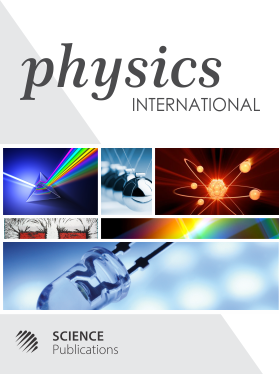Frequency: Continuous
ISSN: 1948-9803 (Print)
ISSN: 1948-9811 (Online)
Research Article Open Access

# BOOM: Rainbow’s Gravity as the Source of Light Refraction

Mohsen Lutephy1
• 1 Department of Chemistry, Islamic Azad University (IAU), Tehran, Iran

## Abstract

On the fully variable light speed (VSL) universe derived by alliance of Mach inertia principle and Planck’s quantized natural units and generalized Minkowski metric, it is extracted the fundamental equation of variable light speed called here Alpha Genesis Prime. Then the fundamental gravity is defined as a vector to justify the light speed variation accordingly. Gravitational vector via a tangential component does set the light speed to be matched with the Alpha Genesis Prime and according to the Pythagorean Theorem; it has also a component normalized to the light velocity. Interestingly by the gravity divided to the tangent and normal components relative to the light velocity we argue the Snell’s law of the light refraction and we find that dependency of the refractive index to the volume density is not fundamental but fundamentally the refractive index follows gravitational potential in bound quantum systems. The gravity is enhanced in bound quantum systems extended from Femto-scale (Nucleuses and strong nuclear force), Micro-gravity (fundamental rainbow’s gravity), the galaxies and clusters as the large scale bound quantum systems, up to the observable universe which the variable gravitational G is Newtonian (constant G in short cosmic time). The refraction of the light is sourced potentially by Rainbow’s gravity in bound quantum systems which the potential integration domain is limited to the wavelength of the photons. Quantum mechanically the photons are force carrier in the range of their wavelength to enhance gravitational G inasmuch as large that atoms to bend the photons similar to enhanced gravity in the nucleuses in the range of hadron wavelengths. Reestablish of the Newtonian mechanics yields to the fundamental gravity which is identical with the refraction of the light and we find that the mechanical potential of the light’s refraction is the gravity.

Physics International
Volume 12 No. 1, 2021, 11-22

DOI:

Submitted On: 16 July 2021 Published On: 23 December 2021

How to Cite: Lutephy, M. (2021). BOOM: Rainbow’s Gravity as the Source of Light Refraction. Physics International, 12(1), 11-22. https://doi.org/10.3844/pisp.2021.11.22

• 2,614 Views